The PANEL Procedure

First-Differenced Methods for One-Way and Two-Way Models

The first-differenced (FD) estimator is an approach that is used to address the problem of omitted variables in econometrics and statistics by using panel data. The estimator is obtained by running a pooled OLS estimation for a regression of the differenced variables. The specification of the models, along with the estimation of the fixed effects, is the same as that described in the sections One-Way Fixed-Effects Model and Two-Way Fixed-Effects Model. To eliminate the fixed effects, you use first-differenced methods to difference them out instead of using the within transformation. Because the intercept is differenced out, the intercept cannot be estimated by first-differenced methods.

Let i be the cross sections and t be the time periods. The regressors and dependent variables are denoted as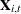and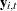, respectively. For the models that have only cross-sectional effects, the data are transformed by first-differencing within each cross section. Therefore, the transformed variables are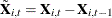for regressors and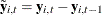for the dependent variable.

For models that have only time effects, the transformation is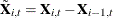for regressors and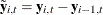for the dependent variable.

For models that have both cross-sectional effects and time effects, the transformation is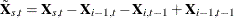for regressors and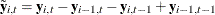for the dependent variable.

The first-differenced estimator is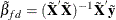The resulting residual can be denoted as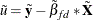. The degree of freedom is the same as in a one-way fixed-effects model or a two-way fixed-effects model when the within transformation is used.

To calculate the predicted value, you can use the previous time period or last individual’s information or both. If the model has only cross-sectional effects, the predicted value is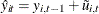. If the model has only time effects, the predicted value is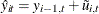. If the model has both cross-sectional and time effects, the predicted value is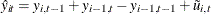.